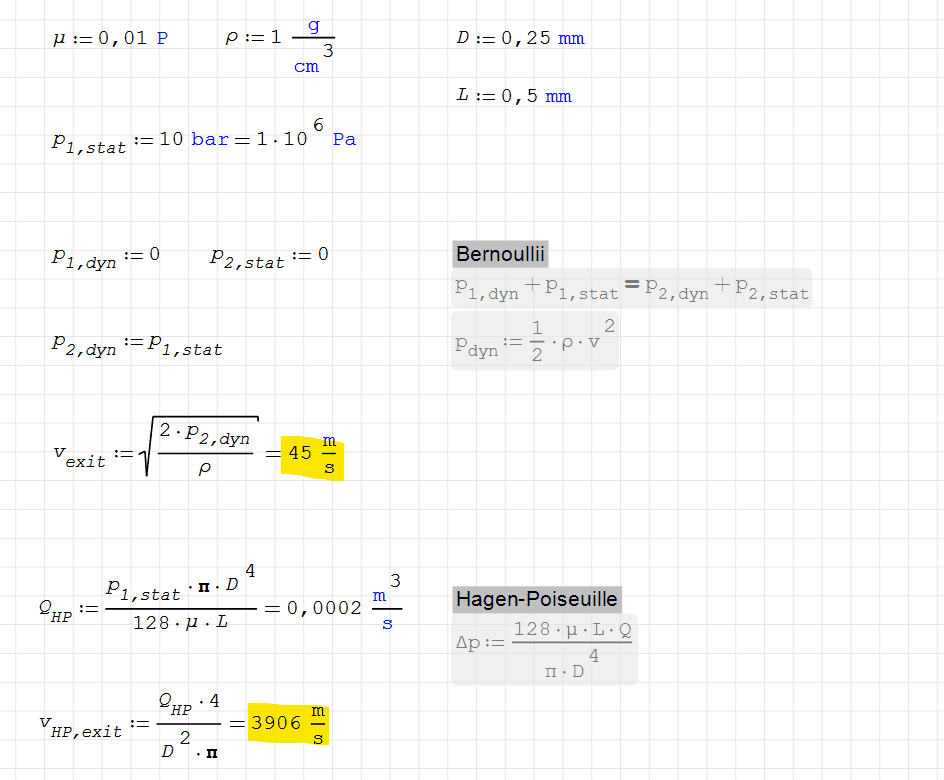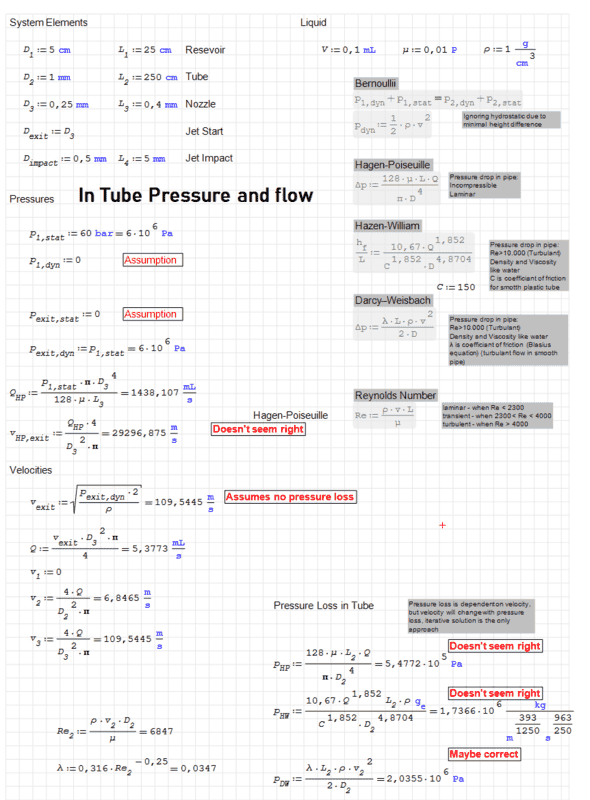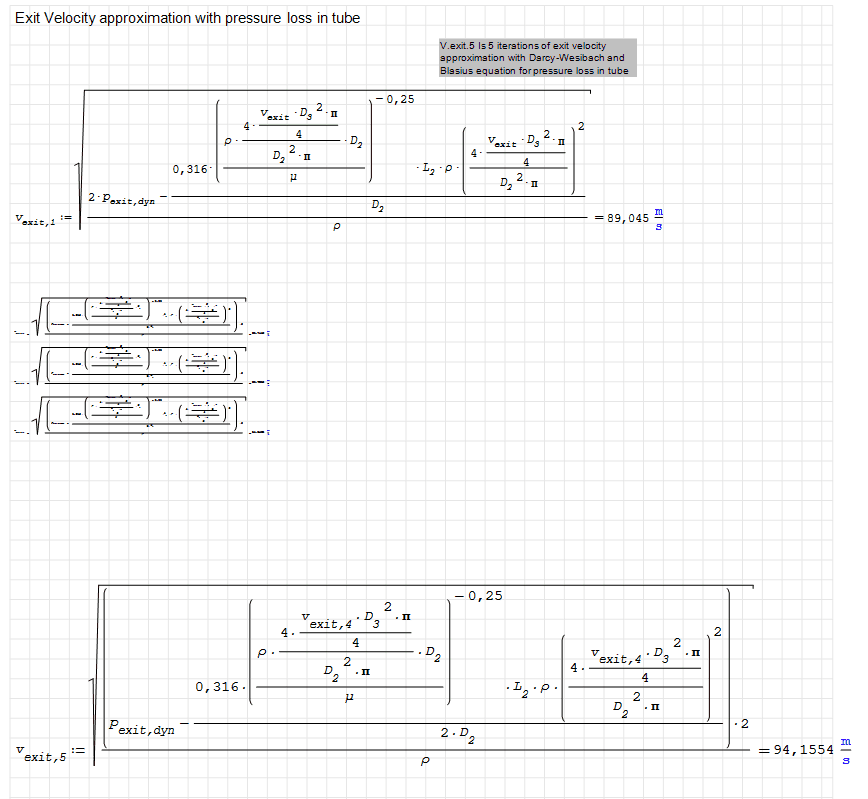# Hagen-Poiseuille vs Bernoulli

bjarke
I am trying to calculate the exit velocity of a nozzle (0,25Ø) that is connected to a high pressure syringe (10 BAR), however I cannot understand why Hagen-Poiseuille will have a higher exit velocity then Bernoulli when HP take viscosity into account.Homework Helper
Hello @bjarke ,!​

Hagen-Poiseuille is for laminar flow. Check the Reynolds number ...

##\ ##

•FluidDroog
Arjan82
Your H-P computation assumes constant velocity. Your Bernoulli computation assumes acceleration from 0 to 45m/s. So your Bernoulli computation also takes the acceleration of mass into account.

•BvU
Arjan82
Actually, I get much more dramatic values for H-P:
Q = 1.9e+4 m3/s, v = 390e3 m/s...

Ps: I said "Bernoulli *also* takes acceleration into account". Actually, Bernoulli has no friction of course so it *only* takes acceleration into account... What you would actually need is a combination of the two (and then you need to check Reynolds as @BvU suggested, otherwise check Moody's Diagram)

•FluidDroog
Homework Helper
Q = 1.9e+4 m3/s,
seems like the math needs checking##\ ##

•Arjan82
Arjan82
millimeters, not meters... 🙈... nvm, I cannot brain today...

bjarke
Hello @bjarke ,!​

Hagen-Poiseuille is for laminar flow. Check the Reynolds number ...

##\ ##
Yeah, with these speeds the Reynolds number is turbulant for both scenarios, but it is my understanding that both HP and bernoulli assumes laminar flow.
But the "pipe" in HP is more an orifice. If I halve the L in HP I would get twice the speed, which doesn't seem right either.
Is there some kind of Diameter to length ratio where HP is no longer accurate?

bjarke
This is the same problem, but expanded for my entire system.
I use the Darcy-Weisbach equation to figure out what my pressure loss is in my tube, and then I iterate this pressure loss into my bernoulli speed to approximate an exit velocity. v=sqrt((2P-static-2P-headloss)/rho)
Does this exit velocity of 94m/s make any sense?

Another problem is that the pressure loss (P-DW) is very close to, and almost ends up being larger than the initial static pressure. So that if I decrease the tube diamater (D2) the loss will be greater, and the resulting velocity will end up being an imaginary numberArjan82
Yeah, with these speeds the Reynolds number is turbulant for both scenarios, but it is my understanding that both HP and bernoulli assumes laminar flow.
No, H-P assumes laminar flow indeed. Bernoulli assumes inviscid flow (i.e. no friction at all)

Mentor
This problem calls for the use of the version of the Bernoulli equation which includes the frictional energy dissipation. Both the acceleration and the frictional drag are going to contribute to the overall pressure drop. The main frictional resistance is going to reside in the 1 mm tube rather than the nozzle. And the flow in the tube is going to be turbulent.

As best I can estimate, the pressure drop in the 1 mm tube is going to be about 0.4 Bars, so most of the pressure drop is going to be due to the acceleration in the nozzle.

•Arjan82
bjarke
This problem calls for the use of the version of the Bernoulli equation which includes the frictional energy dissipation. Both the acceleration and the frictional drag are going to contribute to the overall pressure drop. The main frictional resistance is going to reside in the 1 mm tube rather than the nozzle. And the flow in the tube is going to be turbulent.

As best I can estimate, the pressure drop in the 1 mm tube is going to be about 0.4 Bars, so most of the pressure drop is going to be due to the acceleration in the nozzle.
That sounds much more reasonable than the 20 bar I got. What did you equation look like?

Mentor
That sounds much more reasonable than the 20 bar I got. What did you equation look like?
Well, I started with the 45 m/s you got using Bernoulli without friction. From that, I estimate a velocity of 45/16 = 2.8 m/s in the 1 mm tube. From that, I got a Re of about 2800 in the 1 mm tube, which is just beyond the HP region. I then used Darcy Weissbach to get the pressure drop in the tube of about 0.47 Bars. So the actual acceleration pressure change would only be about 9.5 Bars, rather than 10 Bars. So then, one could continue to iterate until the sum of the pressure drops due to friction plus acceleration is 10 Bars.